• 主成分分析算法的MATLAB代码实现，可以帮助理解主成分分析算法的计算过程，比直接调用MATLAB函数更利于学习。
• PCA主成分分析实现方法Matlab；详细请查看博客资料：http://blog.csdn.net/guyuealian/article/details/68487833
• 主成份分析（PCA）中关于协方差矩阵实现的两种方法MATLAB代码详解PCA
• 用简单易懂的方法讲解了主成分分析的原理，并给出了MATLAB实现程序主成分分析
• 关于主成分分析，内含数据源，代码。且matlab代码解释非常详细，结果易懂
• 这个程序代码很可靠哦，可以直接用matlab实现操作
• 主成分分析法 求矩阵的权重 clc clear close all R = ... [ 0.12 0.41 0.33 0.12 0.03; ... 0.14 0.52 0.21 0.10 0.03; ... 0.18 0.38 0.34 0.07 .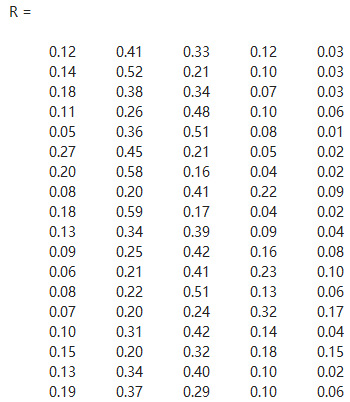主成分分析法 求矩阵的权重

clc
clear
close all
R = ...
[ 0.12          0.41          0.33          0.12          0.03; ...
0.14          0.52          0.21          0.10          0.03; ...
0.18          0.38          0.34          0.07          0.03; ...
0.11          0.26          0.48          0.10          0.06; ...
0.05          0.36          0.51          0.08          0.01; ...
0.27          0.45          0.21          0.05          0.02; ...
0.20          0.58          0.16          0.04          0.02; ...
0.08          0.20          0.41          0.22          0.09; ...
0.18          0.59          0.17          0.04          0.02; ...
0.13          0.34          0.39          0.09          0.04; ...
0.09          0.25          0.42          0.16          0.08; ...
0.06          0.21          0.41          0.23          0.10; ...
0.08          0.22          0.51          0.13          0.06; ...
0.07          0.20          0.24          0.32          0.17; ...
0.10          0.31          0.42          0.14          0.04; ...
0.15          0.20          0.32          0.18          0.15; ...
0.13          0.34          0.40          0.10          0.02; ...
0.19          0.37          0.29          0.10          0.06];

%       Rxx = cov(R); %计算协方差矩阵
mean_R = repmat(mean(R),size(R,1),1)
R_tmp = R - mean_R;

Rxx_tmp = (R_tmp' * R_tmp) / ( size(R_tmp,1) - 1 );%计算协方差矩阵

[Evec Eval] = eig( Rxx_tmp );

%选取特征值较大的特征矢量作为主模式矢量
N_vec = 5;%主模式矢量个数
ModeVec = Evec(: , end - N_vec +1 : end);
DM = R_tmp * ModeVec%在各个模式矢量上投影，实现降维

figure;
plot( sum(abs(DM)) );

sum( sum( R - (DM * ModeVec' + mean_R) ) )%恢复数据验证

展开全文matlab C++
• 应用主成分分析法求指标权重 目录前言一、主成分分析法简单介绍二、如何使用主成分分析法求指标权重？三、源代码四、案例分析五，总结 前言 本文章提供了利用主成分分析法求指标权重的源程序以及一个小小的案例...

应用主成分分析法求指标权重

前言

本文章提供了利用主成分分析法求指标权重的源程序以及一个小小的案例分析，供大家参考。

提示：有关主成分的原理部分这里不再做过多的介绍，csdn上有很多相关内容供大家参考。

一、主成分分析法简单介绍

主成分分析，一种常用的数据降维方法，在保持尽可能多的过程信息变化量的情况下，对相互之间存在相关性的变量所组成的数集进行降维，以获得相互之间互不相关的特征信号的过程，即用较少维的主元信号表征过程数据矩阵的动态变化。

二、如何使用主成分分析法求指标权重？

值得一提的是，该文中提到的各指标在主成分线性组合中的系数，对应着相关系数矩阵的特征向量，如下图所示。三、源代码

代码如下（示例）：

function [W,Com_num] = PCA(A)
%% 数据标准化处理
a = size(A,1);
b = size(A,2);
for i = 1:b
SA(:,i) = (A(:,i) - mean(A(:,i)))/std(A(:,i));
end
%% 计算相关系数矩阵的特征值和特征向量
CM = corrcoef(SA);                          %计算相关系数矩阵
[V,D] = eig(CM);                            %计算特征值和特征向量
for j = 1:b
DS(j,1)=D(b+1-j,b+1-j);                 %对特征值按降序排列
end
for i = 1:b
DS(i,2) = DS(i,1)/sum(DS(:,1));         %贡献率
DS(i,3) = sum(DS(1:i,1))/sum(DS(:,1));  %累计贡献率
end
%% 选择主成分及对应的特征向量
T = 0.9;    %主成分保留率
for K = 1:b
if DS(K,3) >= T
Com_num = K;
break
end
end
%% 提取主成分对应的特征向量
for j = 1:Com_num
PV(:,j)=V(:,b+1-j);       %PV是各指标在各个主成分线性组合中的系数
end
%% 计算各指标权重
w1=PV*DS(1:Com_num,2)/DS(Com_num,3);%对指标在两个主成分线性组合中的系数做加权平均
W=w1/sum(w1);%归一化之后求得各个指标的权重
end

函数的输入A为各指标的数值矩阵，输出值中W为各指标的权重，Com_num为保留的主成分个数。
(注：代码基于https://www.pianshen.com/article/6398423334/修改。)

四、案例分析

应用主成分分析方法求油色谱分析中各种气体的重要程度，即权重值。
一，原始数据二，权重结果表示氢气，甲烷，乙炔，乙烷，一氧化碳，乙烯的权重分别为0.1668,0.2046,0.0646,0.2109,0.1338,0.2195.Com_num=3，表示提取了三个主成分。

五，总结

原理不懂，直接复制粘贴代码也无妨，祝生活愉快。

展开全文算法 matlab
• PCA是根据特征相关程度即协方差值加上线性变换的原理，得到的变换特征向量对应的特征值代表特征成分贡献值大小。 协方差只对特征之间的线性关系敏感，协方差越大，就越可能被去除，所以PCA只能去掉线性相关特征。PCA
• 主成分分析法PCA——MATLAB实现

万次阅读 多人点赞 2019-02-20 00:34:53
1.基本原理 将原来众多具有一定相关性的变量，重新组合为一组新的相互无关的综合变量来代替原来的...6）依据主成分得分的数据，进一步对问题进行后续的分析和建模（主成分回归、变量子集合的选择、综合评价等） ...

1.基本原理

将原来众多具有一定相关性的变量，重新组合为一组新的相互无关的综合变量来代替原来的变量

2.方法步骤

1）对原始数据进行标准化处理

2）计算样本相关系数矩阵

3）计算相关系数矩阵R的特征值和相应的特征向量

4）选择重要的主成分，并写出主成分表达式

5）计算主成分得分

6）依据主成分得分的数据，进一步对问题进行后续的分析和建模（主成分回归、变量子集合的选择、综合评价等）

3.参考代码

%% 数据导入处理
clc
clear all
%% 数据标准化处理
a = size(A,1);
b = size(A,2);
for i = 1:b
SA(:,i) = (A(:,i) - mean(A(:,i)))/std(A(:,i));
end
%% 计算相关系数矩阵的特征值和特征向量
CM = corrcoef(SA);                          %计算相关系数矩阵
[V,D] = eig(CM);                            %计算特征值和特征向量

for j = 1:b
DS(j,1)=D(b+1-j,b+1-j);                 %对特征值按降序排列
end
for i = 1:b
DS(i,2) = DS(i,1)/sum(DS(:,1));         %贡献率
DS(i,3) = sum(DS(1:i,1))/sum(DS(:,1));  %累计贡献率
end

%% 选择主成分及对应的特征向量
T = 0.9;    %主成分保留率
for K = 1:b
if DS(K,3) >= T
Com_num = K;
break
end
end
%% 提取主成分对应的特征向量
for j = 1:Com_num
PV(:,j)=V(:,b+1-j);
end
%% 计算个评价对象的主成分的分
new_score = SA*PV;
for i = 1:a
total_score(i,1)= sum(new_score(i,:));
total_score(i,2)= i;
end
result_report = [new_score,total_score];    %将各主成分的分与总分放在同一个举证中
result_report = sortrows(result_report,-4); %将总分降序排列
%% 输出模型及结果报告
disp('特征值及其贡献率、累计贡献率:')
DS
disp('信息保留率T对应的主成分与特征向量:')
Com_num
PV
disp('主成分的分及排序（按第四列的总分进行降序排列，前3列为各主成分得分，第五列为企业编号）')
result_report

原始数据：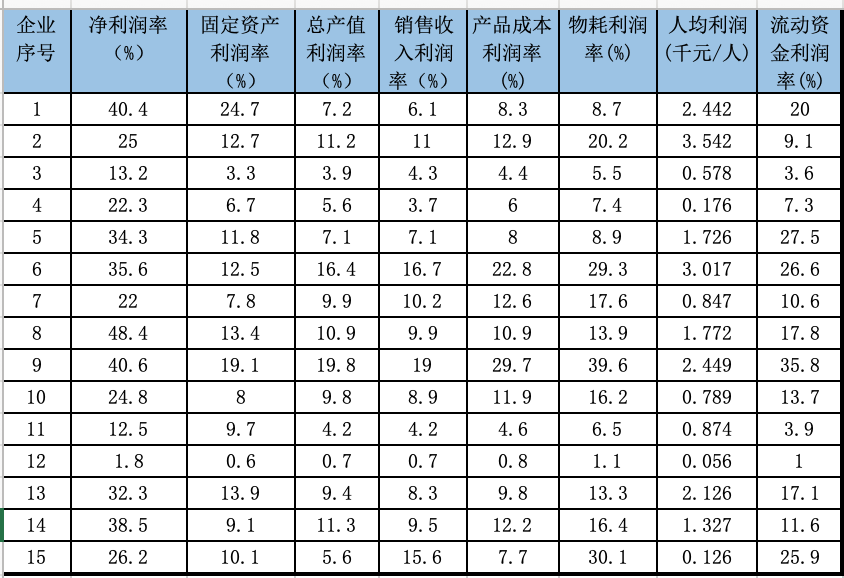运行结果：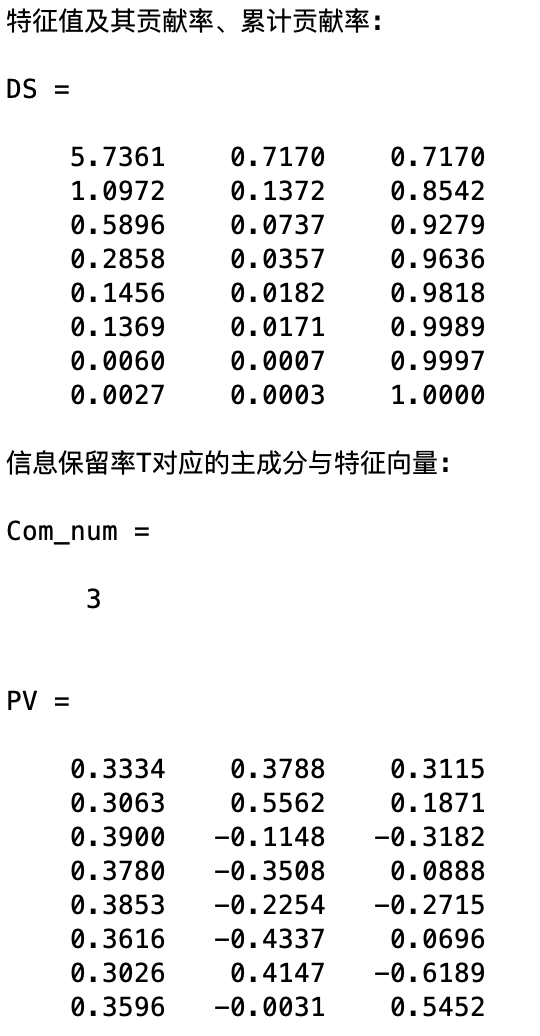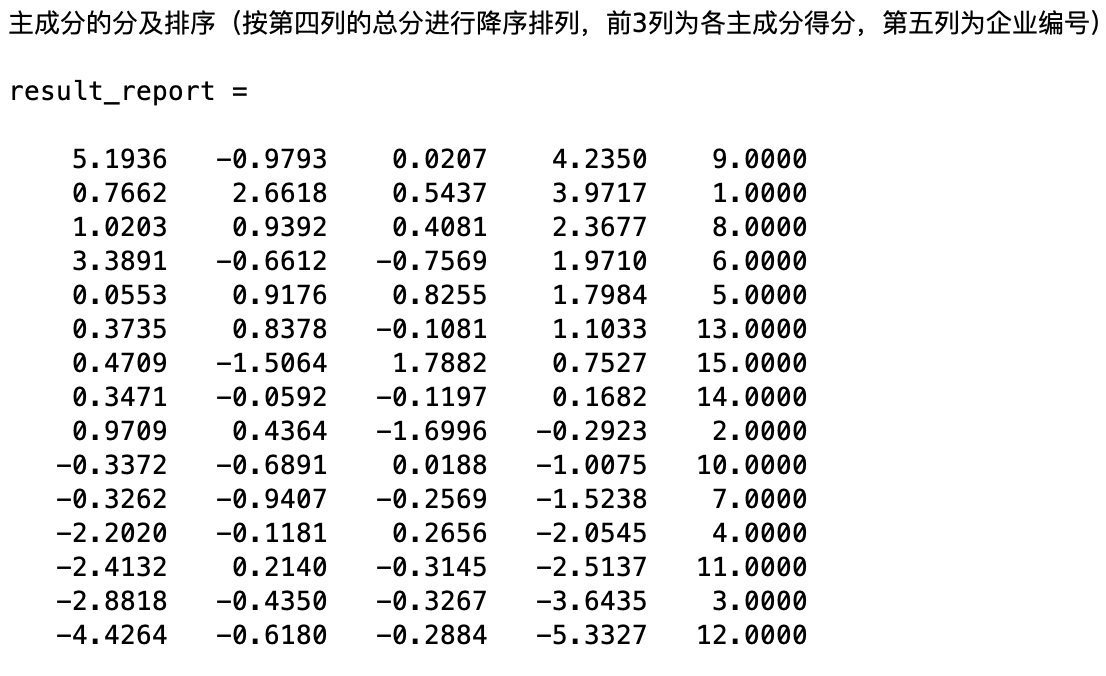展开全文PCA 主成分分析法 matlab
• 主成分分析法原理与MATLAB实现

万次阅读 多人点赞 2016-06-14 19:51:12
主成分分析法是利用降维的思想，把多指标转化为少数几个综合指标（即主成分），其中每个主成分都能够反映原始变量的大部分信息，且所含信息互不重复。这种方法在引进多方面变量的同时将复杂因素归结为几个主成分，使...

1：主成分分析原理：

主成分分析法是利用降维的思想，把多指标转化为少数几个综合指标（即主成分），其中每个主成分都能够反映原始变量的大部分信息，且所含信息互不重复。这种方法在引进多方面变量的同时将复杂因素归结为几个主成分，使问题简单化，同时得到的结果更加科学有效的数据信息。例如，做一件上衣需要测量很多尺寸，如身高，袖长，腰围，胸围，肩宽等十几项指标，但是厂家不可能把尺寸型号分这么多，而是从这十几种指标中综合成几个少数的综合指标作为分类型号，例如综合成反映身高，反映胖瘦和反应特体的三项指标，这就是主成分的思想。主要的方法有特征值分解，SVD(奇异值分解)和NMF(非负矩阵分解)；

2：步骤：

参考：http://wiki.mbalib.com/wiki/%E4%B8%BB%E6%88%90%E5%88%86%E5%88%86%E6%9E%90%E6%B3%95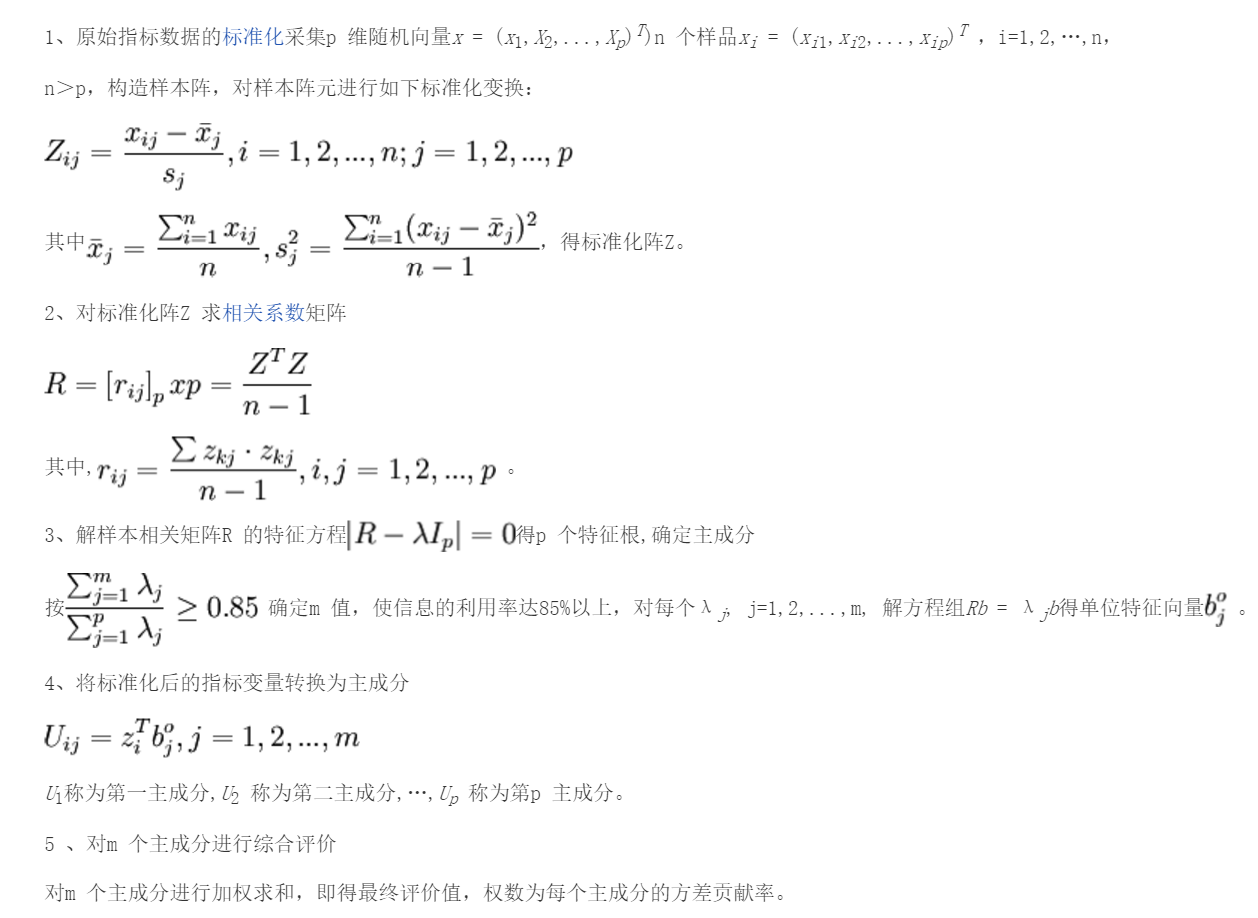第一步采用的是中心化标准化，即元素值减去均值除以标准差，得到的结果服从标准正态分布；

3：matlab代码实现：

x=[1.2,3,-1.1,17;
1.5,5,-3,22;
1.3,4.0,-2,19;
0.7,3,-2.3,11
1,4,-1.2,20.8];
stdr=std(x);                %求各变量的标准差；
[n,m]=size(x);               %矩阵的行与列
sddata=x./stdr(ones(n,1),:);         %标准化变换
[p,princ,egenvalue]=princomp(sddata);  %调用主成分
p=p(:,1:3);                          %输出前3主成分系数；
sc=princ(:,1:3);                       %前3主成分量；
egenvalue;                              %相关系数矩阵的特征值，即各主成分所占比例；
per=100*egenvalue/sum(egenvalue);       %各个主成分所占百分比；

运行结果：

前3主成分系数：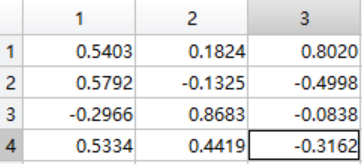特征值矩阵：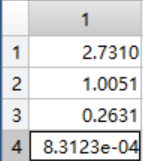百分比：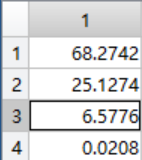所以前3主成分就是：z1=0.5403x1+0.5792x2-0.2966x3+0.5334x4;  z2=0.1824x1-0.1325x2+0.8686x3+0.4419x4;  z3=0.802x1-0.4998x2-0.0838x3-0.3162x4;

上述数据就可以按照这前3主成分进行描述了。

展开全文matlab 主成分分析
• 主成分分析法原理及matlab代码Kmeans聚类 这是 [Coursera Machine Learning] () 课程的第 7 周作业。 概括 在该程序的第一部分中，实现了 K-means 聚类算法并将其应用于压缩图像。 在第二部分中，进行主成分分析以...
• 利用Matlab 编程实现主成分分析1.概述Matlab 语言是当今国际上科学界 (尤其是自动控制领域) 最具影响力、也是最有活力的软件。它起源于矩阵运算，并已经发展成一种高度集成的计算机语言。它提供了强大的科学运算、...
•线性代数 matlab
• 核主成分分析法matlab源代码

热门讨论 2011-11-23 10:07:12
主成分分析法 matlab源代码，非常好的一个例子
• 软件版本声明： Matlab版本：MATLAB R2016a Python版本：Python 3.6.2 Matlab实现参考自博客：...Matlab有关于主成分分析的函数——pca()函数，可以直接调用该函数完成数据集降维。机器学习 数据分析 数据集降维 主成分分析 PCA
• Matlab主成分分析主要目的方法一：直接调用pac函数1.PCA函数的输入与输出参数2.PCA函数的使用方法方法二： 主要目的 主成分分析（或称主分量分析，principal component analysis）由皮尔逊（Pearson,1901）首先...matlab 算法 数据分析
• Matlab主成分分析法

万次阅读 多人点赞 2018-03-06 11:14:07
主成分分析又称主分量分析，由皮尔逊在1901年首次引入，后来由霍特林在1933年进行了发展。主成分分析是一种通过降维技术把多个变量化为少数几个主成分(即综合变量)的多元统计方法，这些主成分能够反映原始变量的大...
• %3.1_cwstd.m,用总和标准化标准化矩阵function std=cwstd(vector)cwsum=sum(vector,1); %对列求和[a,b]=size(vector); %矩阵大小,a 为行数,b 为列数for i=1:afor j=1:bstd(i,j)= vector(i,j)/cwsum(j);endend% 3.2...
• 主成分分析法matlab实现,实例演示 利用 Matlab 编程实现主成分分析1.概述 Matlab 语言是当今国际上科学界 (尤其是自动控制领域 ) 最具影响力、也是最有活力的软件。它起源于矩阵运算，并已经发展成一种高度集成的...
• 进行高光谱图像处理时的降维程序，修改文件中的读入参数名称即可使用。
• 关于主成分分析matlab代码实现的总结

千次阅读 多人点赞 2019-04-01 10:19:18
转自：... 关于主成分分析matlab代码实现的总结 2018年05月18日 15:51:11 It_BeeCoder 阅读数：3375 ...matlab 主成分分析
• matlab实现主成分回归

千次阅读 2019-09-22 15:30:18
主成分分析参考上篇博客，蹦篇博客介绍多元线性回归及其MATLAB实现。 多元线性回归是一种常用的线性建模方法。如果有两个或两个以上的自变量，就称为多元回归。事实上，一种现象常常是与多个因素相联系的，由多个自...
• 主成分分析就是把原来多个变量划为少数几个综合指标的一种统计分析方法。从数学的角度来看这是一种降维处理技术。本文着重思想，无公式推导1.主成分分析的思想1.1 降维处理1.1.1降维处理的作用降维处理是将高维数据...
• 用matlab实现kpca（核主成分分析法）

万次阅读 多人点赞 2019-05-16 15:50:13
主成分分析法是对非线性的高维数据进行降维的一种方法，理论部分这里就不再介绍了，我是在300×12维的数据上进行降维的，代码如下： data=Untitled; [Xrow, Xcol] = size(data); % Xrow：样本个数 Xcol：样本属性...matlab kpca
• 使用MATLAB实现主成分分析所使用的函数主要是【princomp函数】，本博客主要讲述代码实现及部分关键名词解释，理论部分请读者自行百度。 贡献率：每一维数据对于区分整个数据的贡献，贡献率最大的显然是主成分，第...MATLAB 主成分分析 princomp函数
• matlab实现基于主成分分析的人脸识别 MATLAB代码如下 function varargout = face_recognition(varargin) % FACE_RECOGNITION MATLAB code for face_recognition.fig % FACE_RECOGNITION, by itself, creates a new ...matlab 机器学习
• 根据PCA基本原理自己写的PCA算法实现代码，没有使用封装函数，主成分分析函数代码，基于最原始的主成分分析理论，可与吴恩达机器学习课程配套使用
• 主成分分析法 matlab源代码，非常好的一个例子 核主成分分析法 matlab源代码，非常好的一个例子主成分分析法...

matlab实现主成分分析法matlab 订阅